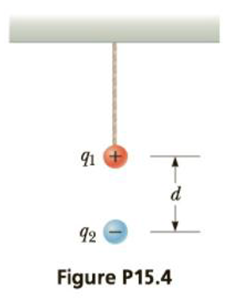Chapter 15, Problem 4P

Chapter
Section
Textbook Problem

A small sphere of mass m = 7.50 g and charge q1 = 32.0 nC is attached to the end of a string and hangs vertically as in Figure P15.4. A second charge of equal mass and charge q2 = –58.0 nC is located below the first charge a distance d = 2.00 cm below the first charge as in Figure P15.4. (a) Find the tension in the string. (b) If the string can withstand a maximum tension of 0.180 N, what is the smallest value d can have before the string breaks?(a)

To determine
The tension in the string.

Explanation

Given info: Mass of the sphere (m) is 7.50 g. The charges q1=32.0nC and q2=58.0nC are separated by a distance d=2.00m .

The tension is equal to the Coulomb force and the weight of the sphere.

Formula to calculate the tension is,

T=mg+ke|q1||q2|d2

• ke is the Coulomb constant.
• g is the acceleration due to gravity.

Substitute 7.50 g for m, 9.8ms2 for g, 8.99×109N.m2/C2 for ke , 32.0nC for q1 , 58.0nC for q2 and 2.00 m for d.

T=(7.50g)(9.8ms2)+[(8

(b)

To determine
The smallest value of d.

Still sussing out bartleby?

Check out a sample textbook solution.

See a sample solution

The Solution to Your Study Problems

Bartleby provides explanations to thousands of textbook problems written by our experts, many with advanced degrees!

Get Started